## Example Questions

### Example Question #1 : How To Add Trinomials

Subtract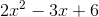from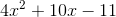.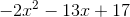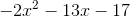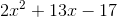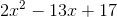Explanation:

Step 1: We need to read the question carefully. It says subtract from. When you see the word "from", you read the question right to left.

I am subtracting the left equation from the right equation.

Step 2: We need to write the equation on the right minus the equation of the left.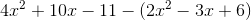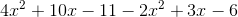Step 4: Combine like terms: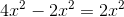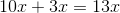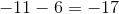Step 5: Put all the terms together, starting with highest degree. The degree of the terms is the exponent. Here, the highest degree is 2 and lowest is zero.

The final equation is